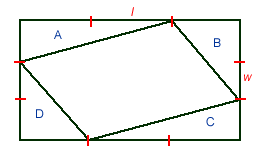#### You may also like### Kissing Triangles

Determine the total shaded area of the 'kissing triangles'.### Isosceles

Prove that a triangle with sides of length 5, 5 and 6 has the same area as a triangle with sides of length 5, 5 and 8. Find other pairs of non-congruent isosceles triangles which have equal areas.Four rods, two of length a and two of length b, are linked to form a kite. The linkage is moveable so that the angles change. What is the maximum area of the kite?

# Rati-o

##### Age 11 to 14 Challenge Level:

Correct solutions were received from Chen from The Chinese High School Singapore, Andrei of School 205 Bucharest, Mary of Brirchwood High School (whose solutions is used as the basis of the one below) and Sana, Jenny, Chris and Rosion of Madras College, St. Andrews.l is a 1/3 the length of the rectangle

w is a1/3 the width of the rectangle

area of the rectangle = 3l x 3w = 9lw

Area triangle A = Area of triangle C = lw

Area triangle B = Area of triangle D= lw

Therefore area of the parallelogram = 9lw - 4lw = 5lw

Therefore ratio of the area of the parallelogram to the area of the rectangle is 5lw : 9lw = 5:9